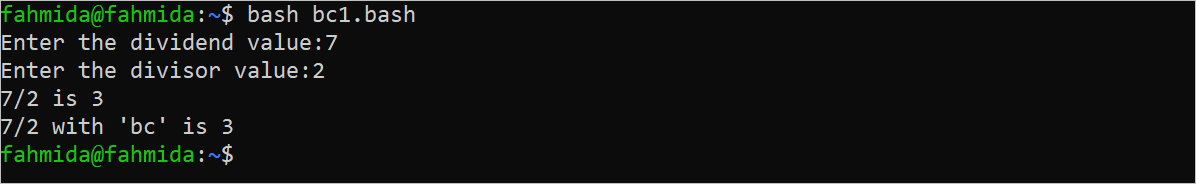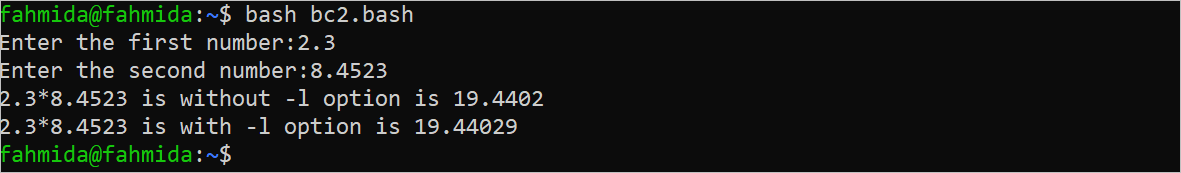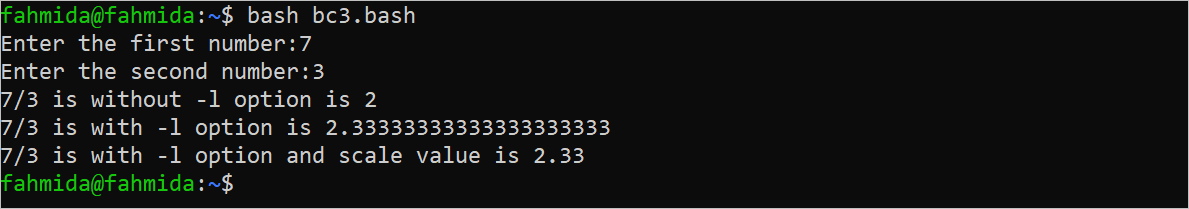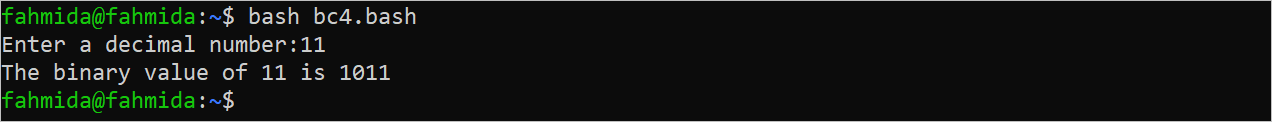| by Arround The Web | No comments

## Bc Command in Bash Scripts

One of the major limitations of Bash is that it can’t calculate the fractional value of the arithmetic operations like other programming languages. The Bash “bc” command is required to solve this issue. The full form of “bc” is “basic calculator”. The fractional value of any mathematical calculation can be generated with the precision value using this command. Different uses of the “bc” command in Bash are shown in this tutorial.

Syntax:

The syntax of the “bc” command is given as follows:

bc [options] [ file ]

This command can be used without any option. Different types of options can be used with this command to perform different mathematical tasks. The file can be used with the “bc” command to apply it to the content of the file which is also optional.

## Different Uses of the “Bc” Command

The uses of the “bc” command without any option and with an option are shown in this part of the tutorial.

### Example 1: Simple Use of the “Bc” Command

Create a Bash file with the following script that uses the “bc” command without any option. Two numeric values are taken as input and the division result of these numbers with and without the “bc” command are printed:

#!/bin/bash

#Take two input values

echo -n "Enter the dividend value:"

echo -n "Enter the divisor value:"

#Calculate the division without the 'bc' command

((result=dividend/divisor))

echo "\$dividend/\$divisor is \$result"

#Calculate the division with the 'bc' command

((result=dividend/divisor | bc))

echo "\$dividend/\$divisor with 'bc' is \$result"

The following output appears after executing the script. According to the output, the appropriate division result is not returned by the “bc” command without any option:### Example 2: Using “Bc” with the -L Option

The –l option is used with the “bc” command to calculate the appropriate result of the arithmetic operation that returns the fractional value. Create a Bash file with the following script that uses the “bc” command without any option and with the -l option. Two numeric values are taken as input and the multiplication result of these numbers with the “bc” command are printed.

#!/bin/bash

#Take two input values

echo -n "Enter the first number:"

echo -n "Enter the second number:"

#Calculate the multiplication of two numbers using the 'bc' command

echo -n "\$n1*\$n2 is without -l option is "

echo \$n1*\$n2 | bc

echo -n "\$n1*\$n2 is with -l option is "

echo \$n1*\$n2 | bc -l

The following output appears after executing the script. According to the output, the appropriate multiplication result is returned by the “bc” command with the -l option:### Example 3: Using “Bc” with the -L Option and Scale Value

The scale value is used to format the output of the “bc” command with the particular digits after the decimal point. Create a Bash file with the following script that uses the “bc” command without any option and with the -l option. The scale value with 2 is used to format the fractional output. Two numeric values are taken as input and the division result of these numbers with the “bc” command is printed:

#!/bin/bash

#Take two input values

echo -n "Enter the first number:"

echo -n "Enter the second number:"

#Calculate the multiplication of two numbers using the 'bc' command

echo -n "\$n1/\$n2 is without -l option is "

echo \$n1/\$n2 | bc

echo -n "\$n1/\$n2 is with -l option is "

echo \$n1/\$n2 | bc -l

echo -n "\$n1/\$n2 is with -l option and scale value is "

echo "scale=2; \$n1/\$n2" | bc -l

The following output appears after executing the script. The output of the division is formatted to 2 digits after the decimal point using the scale value:### Example 4: Convert the Decimal Number to Binary Using “Bbc”

The obase flag can be used to convert from one number system to another number system. It contains the value from 2 to 999. Create a Bash file with the following script that uses the “bc” command that converts a decimal number into a binary number. The value of the obase is 2 to do this task.

#!/bin/bash

#Take a decimal value

echo -n "Enter a decimal number:"

echo -n "The binary value of \$dec is "

#Convert the decimal value into a binary value

echo "obase=2;\$dec" | bc

The following output appears after executing the script. Eleven (11) is taken as input and the binary value of 11 is 1011 which is printed in the output:## Conclusion

Various uses of the “bc” command are shown in this tutorial using multiple examples. The accurate result of all types of mathematical operations can be achieved using the “bc” command. The basic uses of the “bc” command will be cleared after reading this tutorial.

Source: linuxhint.com